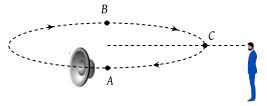A small source of sound moves on a circle as shown in the figure and an observer is standing on O. Let n1, n2 and n3 be the frequencies heard when the source is at A, B and C respectively. Then(1) n1 > n2 > n3

(2) n2 > n3 > n1

(3) n1 = n2 > n3

(4) n2 > n1 > n3

(2) At point A, source is moving away from observer so apparent frequency n1 < n (actual frequency) At point B source is coming towards observer so apparent frequency n2 > n and point C source is moving perpendicular to observer so n3 = n

Hence n2 > n3 > n1

Difficulty Level:

• 19%
• 50%
• 30%
• 3%